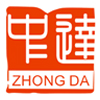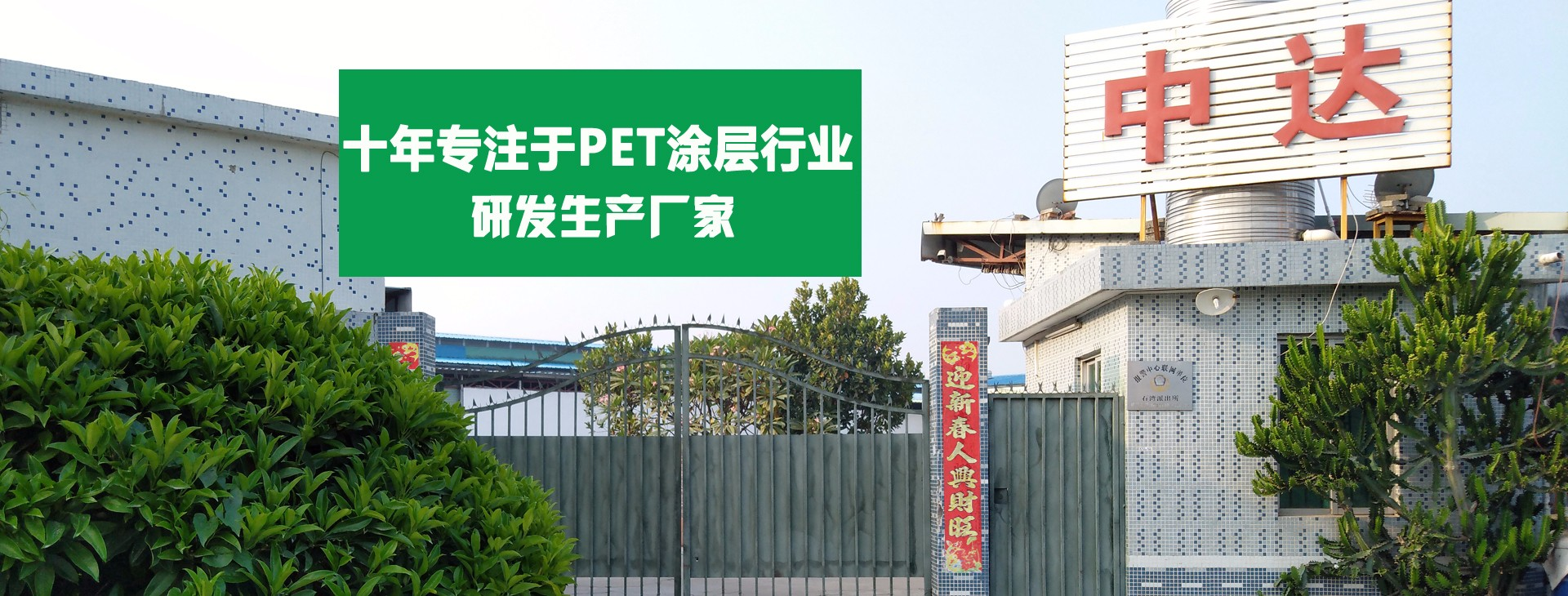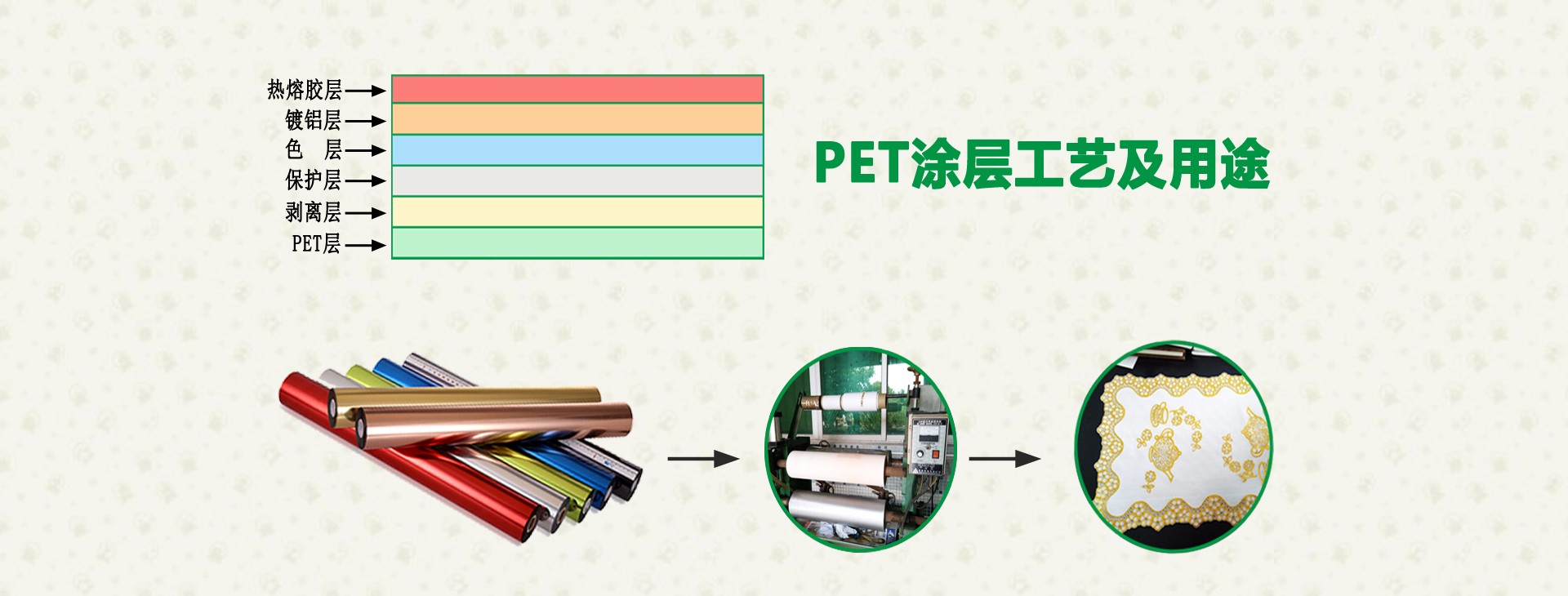••0752-2032327
0752-2032308
0752-6618501(助理专线)红+黄+蓝=黑

红＋黄=橙(红多黄少，俗称桔红)

黄＋蓝＝绿(黄多蓝少)

草绿 蓝绿(蓝多黄少)

深绿 蓝＋红＝紫(红多蓝少)

蓝紫(蓝多红少)

红灰色(红多，黄、蓝少)

黄灰(橙加黄)

黄灰色(黄多，红、蓝少)

蓝灰(绿加紫)

蓝灰色(蓝多，红、黄少)

红灰(橙加紫)

纯灰(黑加白)

绿多+黄少=草绿色

白+黑少=灰

铁红+黑=咖啡色

紫色=红+蓝

草绿=绿多+黄少

哈密瓜黄=绿少+黄多

豆沙色=铁红+黄少

奶茶色=(红45%黄50%蓝5%)或(橙色少加黑，橙95%黑5%)

姜黄色=(氧化铁黄)感觉不够亮，适当加点中黄，姜黄色本身就是高档的颜色，所有黄色都可以加点中黄，提高亮度。

其次，我们调色是根据实际来说的，不可能用数量来表示，练习的时候，用稀释的白漆练习，多试几次。

记住过程，我想这些资料基本够用了。

（红）+（黄）=（橙）

（蓝）+（红）=（紫）

（蓝）+（黄）=（绿）

（红）+（黄）+（蓝）=（黑色）

熟褐色 = 柠檬黄 + 纯黑色（湖蓝）+ 玫瑰红

粉玫瑰红 = 纯白色 + 玫瑰红

朱红色 = 柠檬黄 + 玫瑰红

暗红色 = 玫瑰红 + 纯黑色

紫红色 = 纯紫色 + 玫瑰红

褚石红 = 玫瑰红 + 柠檬黄 + 纯黑色

粉蓝色 = 纯白色 + 天蓝色

蓝绿色 = 草绿色 + 天蓝色

灰蓝色 = 天蓝色 + 纯黑色

浅灰蓝 = 天蓝色 + 纯黑色 + 纯紫色

粉绿色 = 纯白色 + 草绿色

黄绿色 = 柠檬黄 + 草绿色

墨绿色 = 草绿色 + 纯黑色

粉紫色 = 纯白色 + 纯紫色

啡色 = 玫瑰红 + 纯黑色

粉柠檬黄 = 柠檬黄 + 纯白色

藤黄色 = 柠檬黄 + 玫瑰红

桔黄色 = 柠檬黄 + 玫瑰红

土黄色 = 柠檬黄 + 纯黑色 + 玫瑰红

大红+柠檬黄=桔黄
柠檬黄+湖蓝=草绿，加多了湖蓝就是粉绿

大红+湖蓝=紫罗兰，加多了大红就是玫瑰红

大红+桔黄=中黄

大红+草绿=熟褐

大红+紫罗兰=玫瑰红

柠檬黄+紫罗兰=熟褐

柠檬黄+草绿=浅绿

湖蓝+紫罗兰=青莲

假如要颜色变浅，加上白色(可以根据白色的份量的增多逐渐变浅)，假如要颜色变深或者灰，加上黑色(黑色加上一点就能变深)。

在诸多颜色当中你可以大胆地调色，份量的多少，调出的效果不同。

我们提醒您需要记住一个要点： 紫色不能加黄色，绿色不能加红色，蓝色不能加橙色。因为这样的互补色调出来是很深的灰色或者是黑色的。

• 返回顶部
• 0752-2032327
• 在线咨询
• 手机站